# RD Sharma Solutions For Class 12 Maths Exercise 6.1 Chapter 6 Determinants

RD Sharma Solutions for Class 12 Maths Exercise 6.1 Chapter 6 Determinants is provided here. The determinant of a square matrix of order 1, 2, 3 and 4, singular matrix, minors and cofactors of given determinants are the main topics which are explained under exercise 6.1. This exercise can be used as a model of reference by the students to improve their conceptual knowledge and understand the different ways used to solve the problems.

The PDF of RD Sharma Solutions for Class 12 Maths Exercise 6.1 is available here. These RD Sharma Solutions are formulated by BYJU’S experts in Maths to increase students confidence, which plays a crucial role in their board exams.

## RD Sharma Solutions For Class 12 Chapter 6 Determinants Exercise 6.1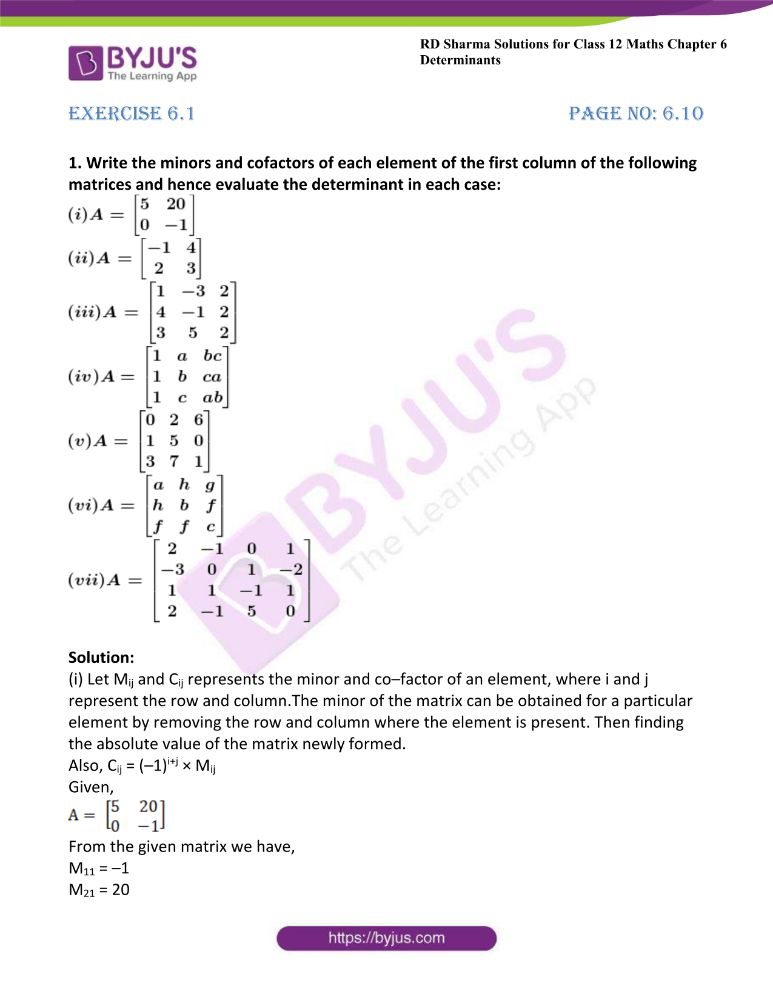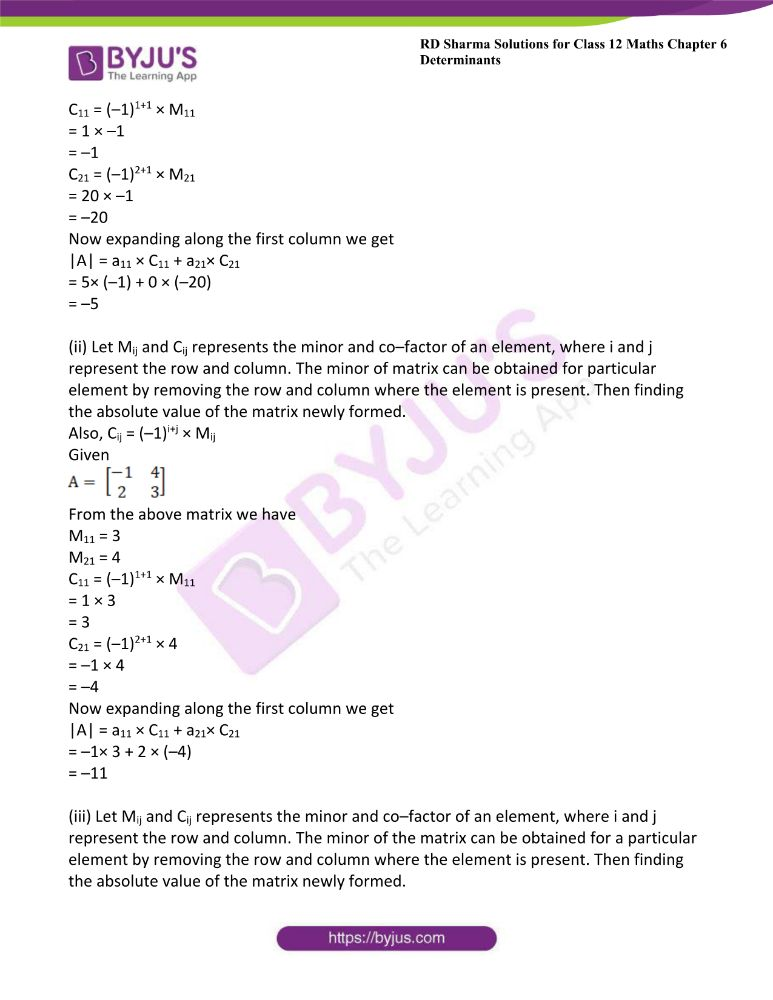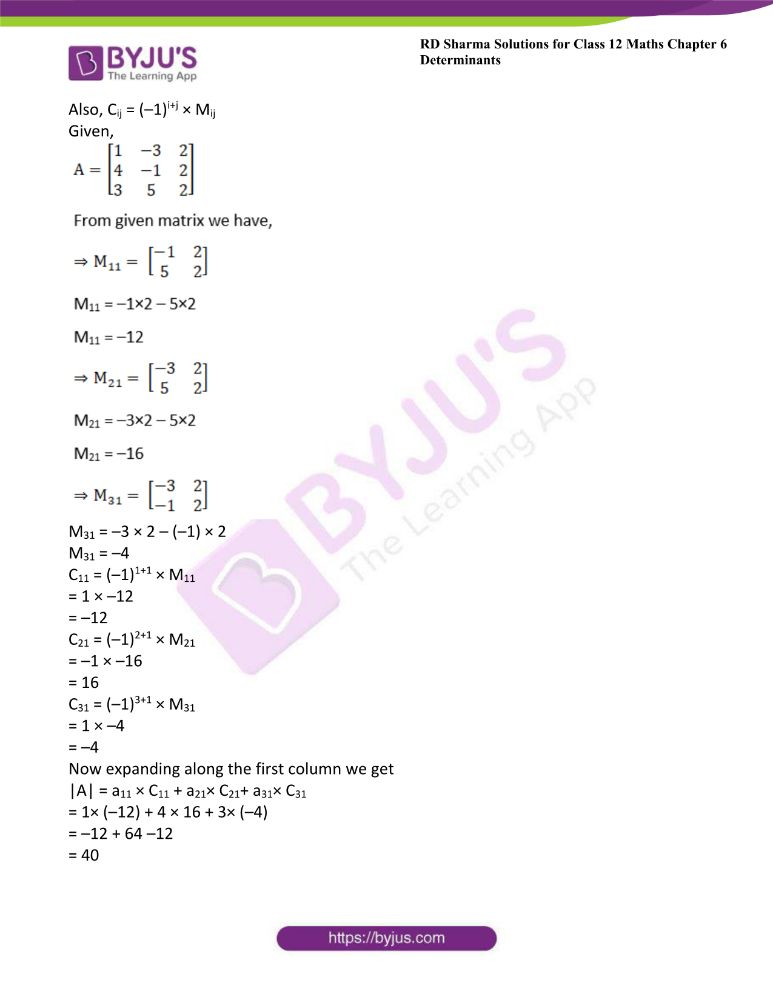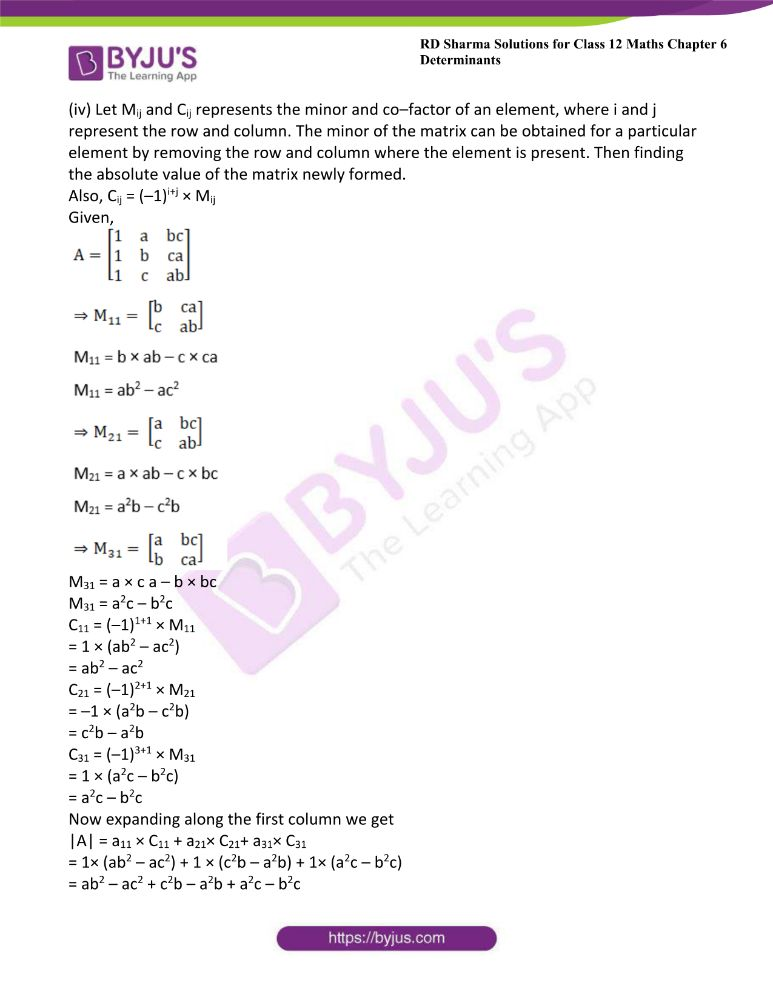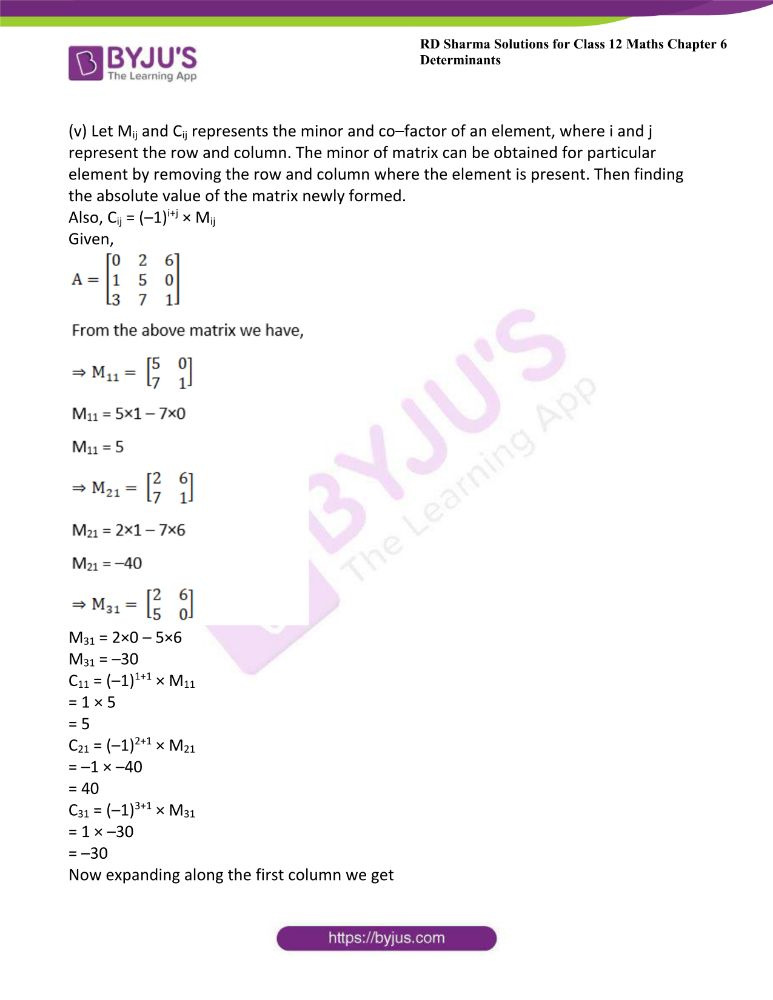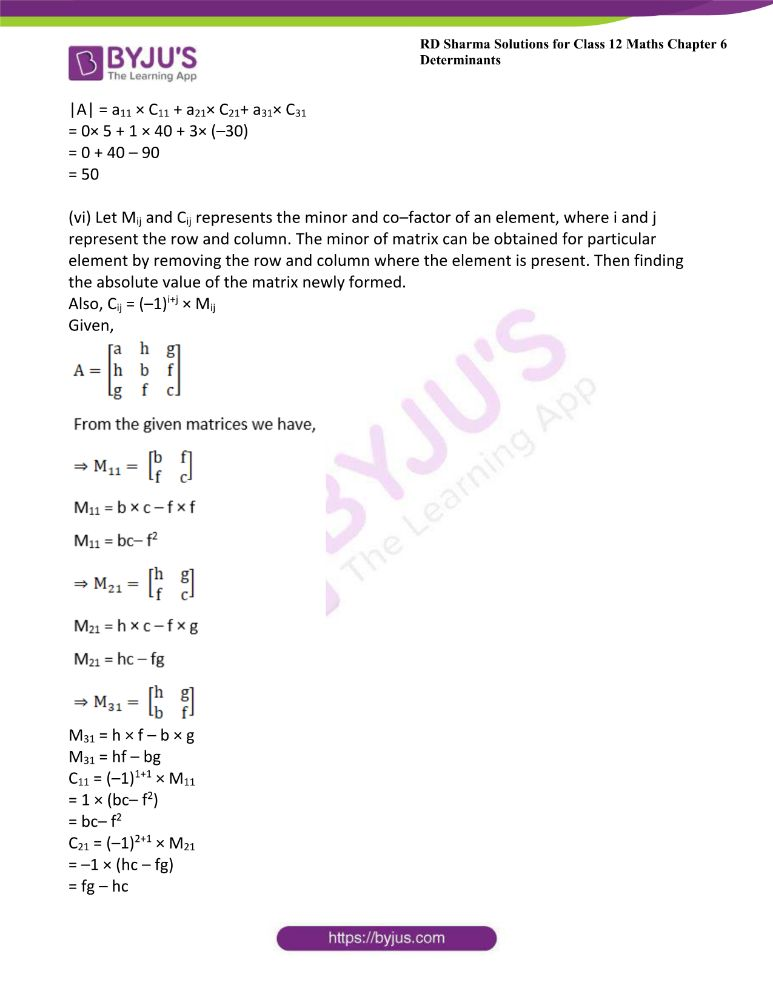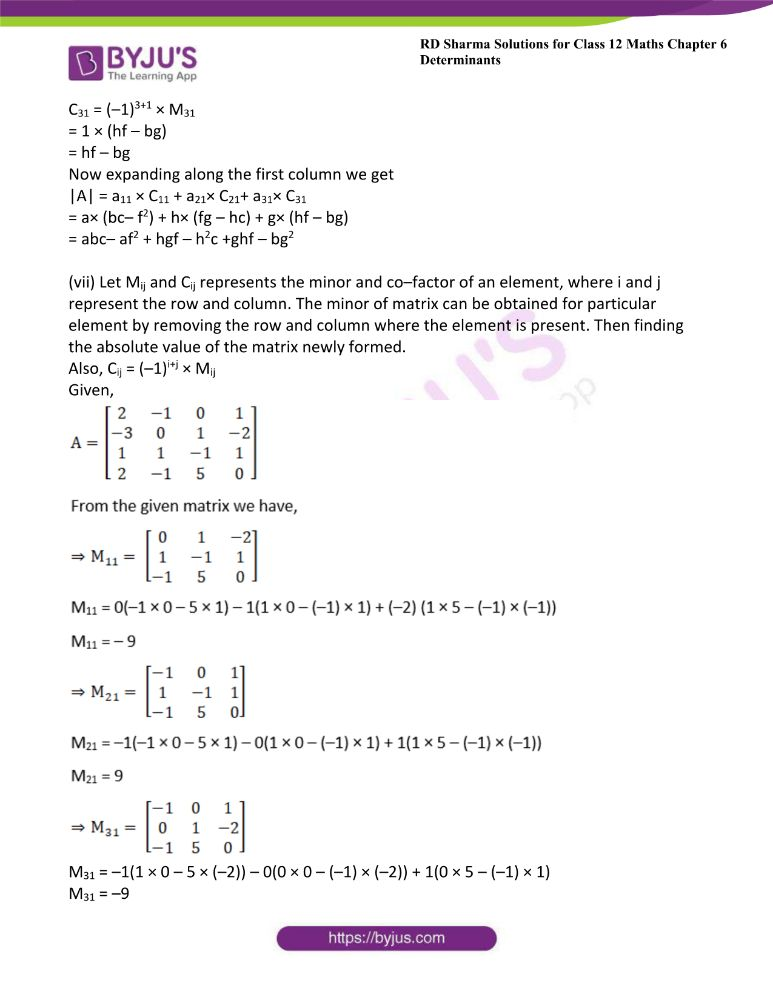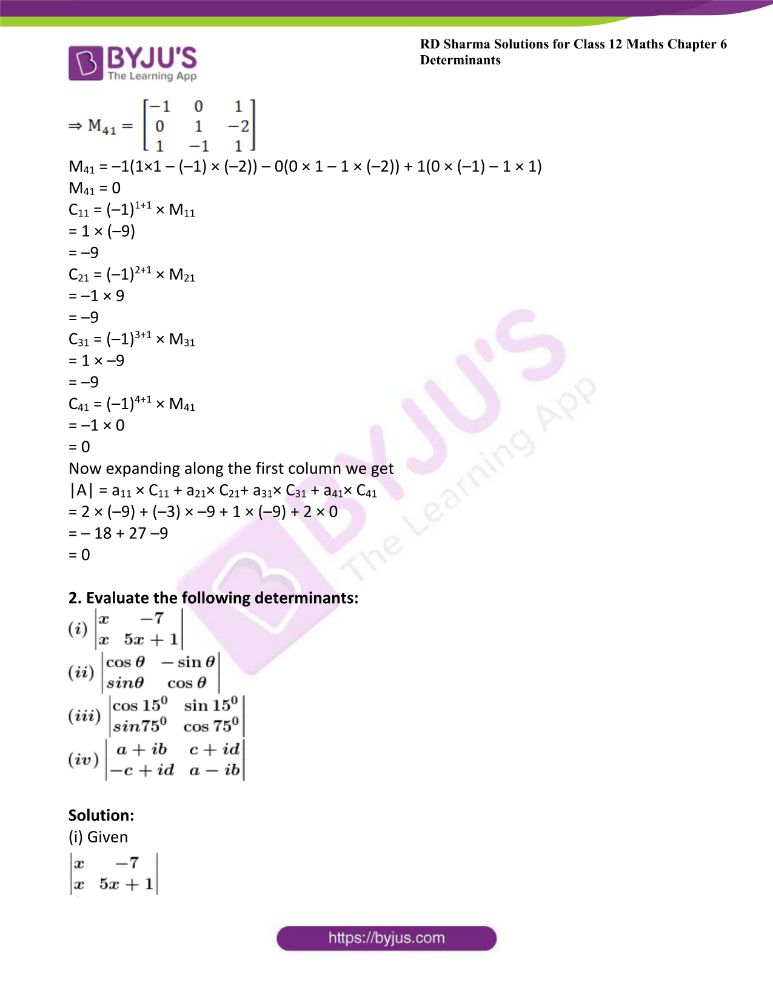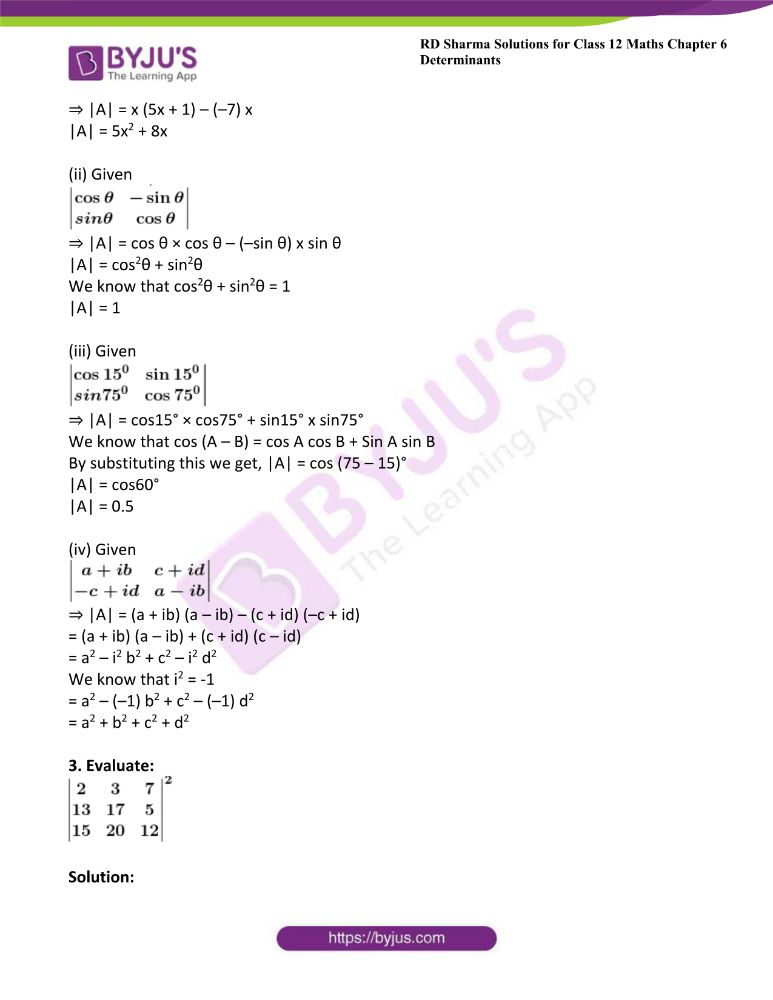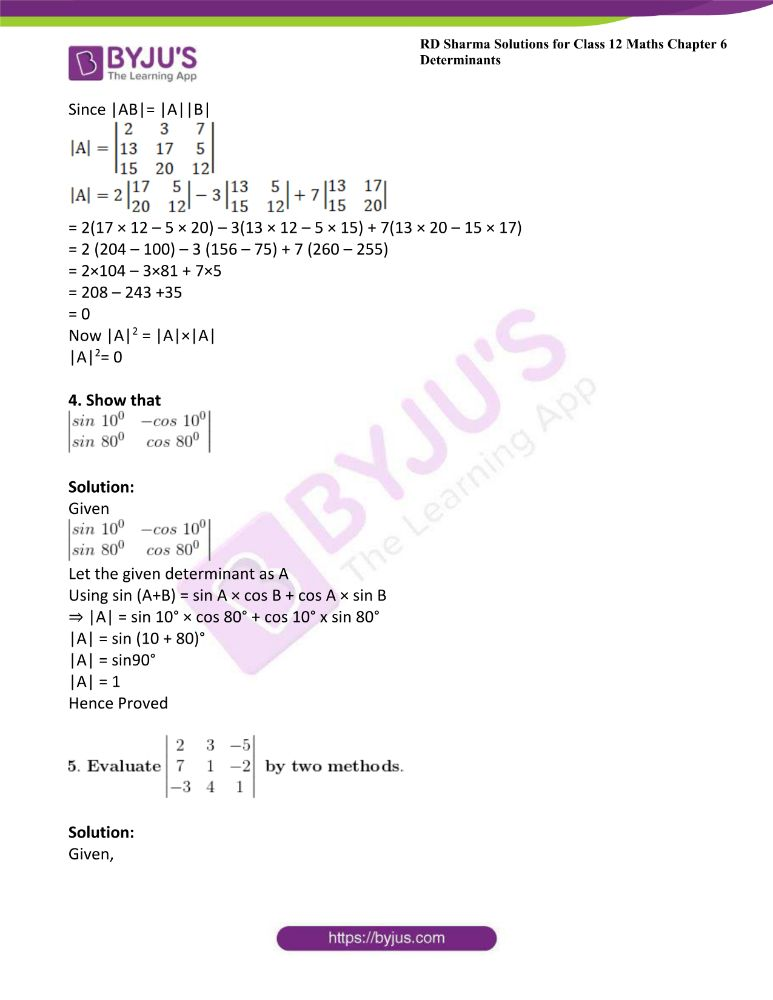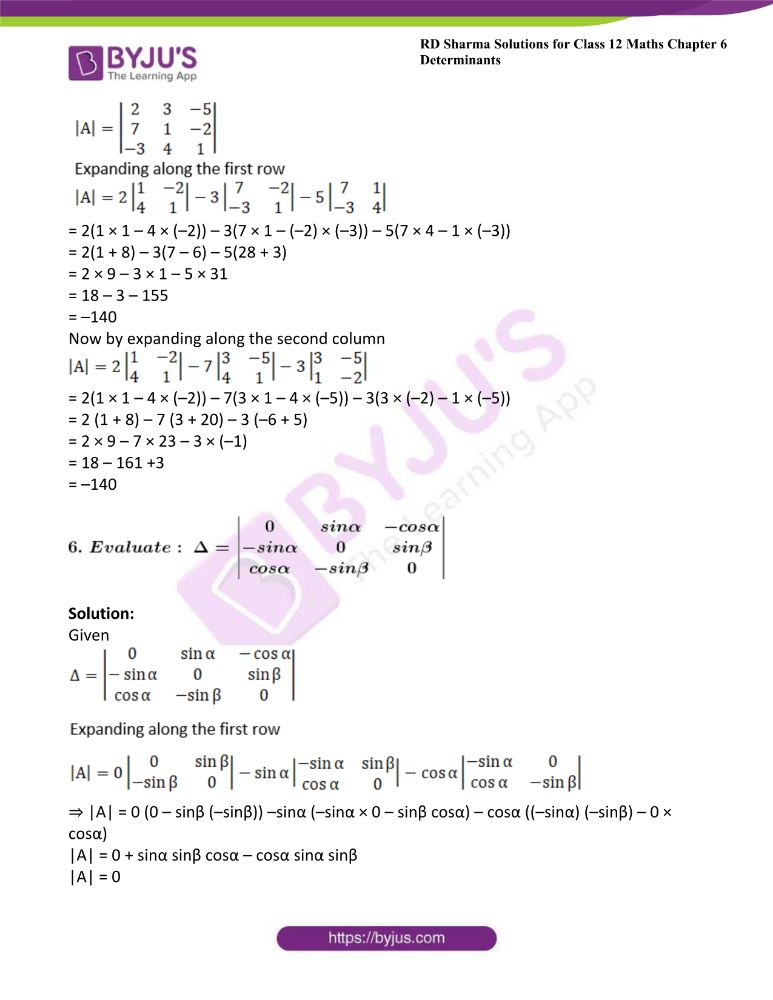### Access other exercises of RD Sharma Solutions For Class 12 Chapter 6 – Determinants

Exercise 6.2 Solutions

Exercise 6.3 Solutions

Exercise 6.4 Solutions

Exercise 6.5 Solutions

### Access answers to Maths RD Sharma Solutions For Class 12 Chapter 6 – Determinants Exercise 6.1

Exercise 6.1 Page No: 6.10

1. Write the minors and cofactors of each element of the first column of the following matrices and hence evaluate the determinant in each case: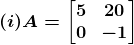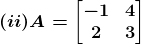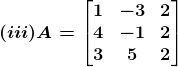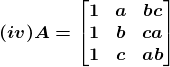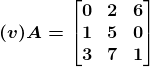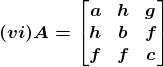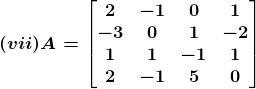Solution:

(i) Let Mij and Cij represents the minor and co–factor of an element, where i and j represent the row and column.The minor of the matrix can be obtained for a particular element by removing the row and column where the element is present. Then finding the absolute value of the matrix newly formed.

Also, Cij = (–1)i+j × Mij

Given,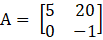From the given matrix we have,

M11 = –1

M21 = 20

C11 = (–1)1+1 × M11

= 1 × –1

= –1

C21 = (–1)2+1 × M21

= 20 × –1

= –20

Now expanding along the first column we get

|A| = a11 × C11 + a21× C21

= 5× (–1) + 0 × (–20)

= –5

(ii) Let Mij and Cij represents the minor and co–factor of an element, where i and j represent the row and column. The minor of matrix can be obtained for particular element by removing the row and column where the element is present. Then finding the absolute value of the matrix newly formed.

Also, Cij = (–1)i+j × Mij

Given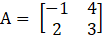From the above matrix we have

M11 = 3

M21 = 4

C11 = (–1)1+1 × M11

= 1 × 3

= 3

C21 = (–1)2+1 × 4

= –1 × 4

= –4

Now expanding along the first column we get

|A| = a11 × C11 + a21× C21

= –1× 3 + 2 × (–4)

= –11

(iii) Let Mij and Cij represents the minor and co–factor of an element, where i and j represent the row and column. The minor of the matrix can be obtained for a particular element by removing the row and column where the element is present. Then finding the absolute value of the matrix newly formed.

Also, Cij = (–1)i+j × Mij

Given,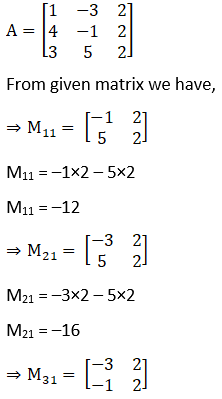M31 = –3 × 2 – (–1) × 2

M31 = –4

C11 = (–1)1+1 × M11

= 1 × –12

= –12

C21 = (–1)2+1 × M21

= –1 × –16

= 16

C31 = (–1)3+1 × M31

= 1 × –4

= –4

Now expanding along the first column we get

|A| = a11 × C11 + a21× C21+ a31× C31

= 1× (–12) + 4 × 16 + 3× (–4)

= –12 + 64 –12

= 40

(iv) Let Mij and Cij represents the minor and co–factor of an element, where i and j represent the row and column. The minor of the matrix can be obtained for a particular element by removing the row and column where the element is present. Then finding the absolute value of the matrix newly formed.

Also, Cij = (–1)i+j × Mij

Given,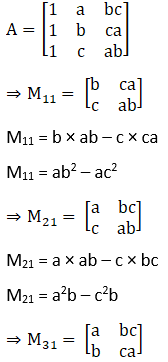M31 = a × c a – b × bc

M31 = a2c – b2c

C11 = (–1)1+1 × M11

= 1 × (ab2 – ac2)

= ab2 – ac2

C21 = (–1)2+1 × M21

= –1 × (a2b – c2b)

= c2b – a2b

C31 = (–1)3+1 × M31

= 1 × (a2c – b2c)

= a2c – b2c

Now expanding along the first column we get

|A| = a11 × C11 + a21× C21+ a31× C31

= 1× (ab2 – ac2) + 1 × (c2b – a2b) + 1× (a2c – b2c)

= ab2 – ac2 + c2b – a2b + a2c – b2c

(v) Let Mij and Cij represents the minor and co–factor of an element, where i and j represent the row and column. The minor of matrix can be obtained for particular element by removing the row and column where the element is present. Then finding the absolute value of the matrix newly formed.

Also, Cij = (–1)i+j × Mij

Given,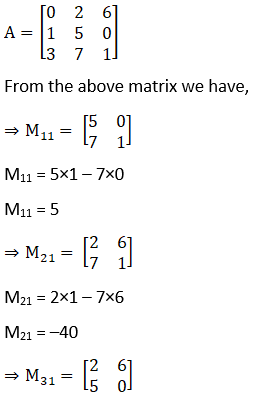M31 = 2×0 – 5×6

M31 = –30

C11 = (–1)1+1 × M11

= 1 × 5

= 5

C21 = (–1)2+1 × M21

= –1 × –40

= 40

C31 = (–1)3+1 × M31

= 1 × –30

= –30

Now expanding along the first column we get

|A| = a11 × C11 + a21× C21+ a31× C31

= 0× 5 + 1 × 40 + 3× (–30)

= 0 + 40 – 90

= 50

(vi) Let Mij and Cij represents the minor and co–factor of an element, where i and j represent the row and column. The minor of matrix can be obtained for particular element by removing the row and column where the element is present. Then finding the absolute value of the matrix newly formed.

Also, Cij = (–1)i+j × Mij

Given,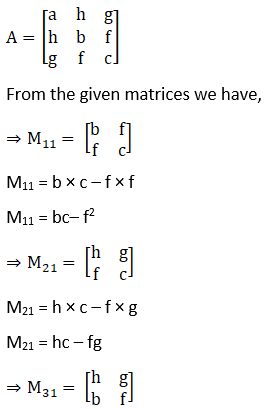M31 = h × f – b × g

M31 = hf – bg

C11 = (–1)1+1 × M11

= 1 × (bc– f2)

= bc– f2

C21 = (–1)2+1 × M21

= –1 × (hc – fg)

= fg – hc

C31 = (–1)3+1 × M31

= 1 × (hf – bg)

= hf – bg

Now expanding along the first column we get

|A| = a11 × C11 + a21× C21+ a31× C31

= a× (bc– f2) + h× (fg – hc) + g× (hf – bg)

= abc– af2 + hgf – h2c +ghf – bg2

(vii) Let Mij and Cij represents the minor and co–factor of an element, where i and j represent the row and column. The minor of matrix can be obtained for particular element by removing the row and column where the element is present. Then finding the absolute value of the matrix newly formed.

Also, Cij = (–1)i+j × Mij

Given,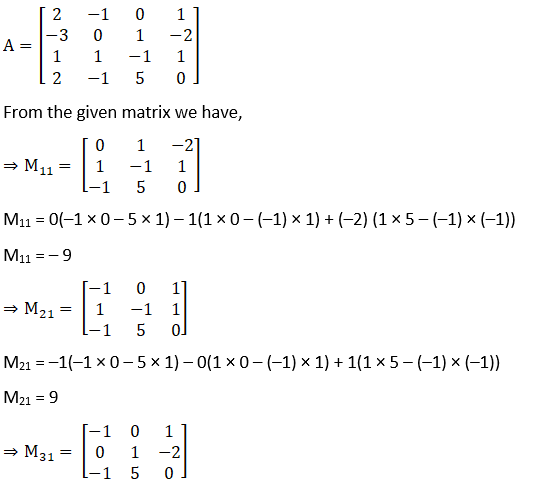M31 = –1(1 × 0 – 5 × (–2)) – 0(0 × 0 – (–1) × (–2)) + 1(0 × 5 – (–1) × 1)

M31 = –9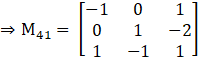M41 = –1(1×1 – (–1) × (–2)) – 0(0 × 1 – 1 × (–2)) + 1(0 × (–1) – 1 × 1)

M41 = 0

C11 = (–1)1+1 × M11

= 1 × (–9)

= –9

C21 = (–1)2+1 × M21

= –1 × 9

= –9

C31 = (–1)3+1 × M31

= 1 × –9

= –9

C41 = (–1)4+1 × M41

= –1 × 0

= 0

Now expanding along the first column we get

|A| = a11 × C11 + a21× C21+ a31× C31 + a41× C41

= 2 × (–9) + (–3) × –9 + 1 × (–9) + 2 × 0

= – 18 + 27 –9

= 0

2. Evaluate the following determinants: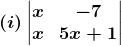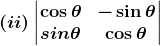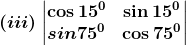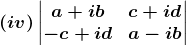Solution:

(i) Given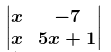⇒ |A| = x (5x + 1) – (–7) x

|A| = 5x2 + 8x

(ii) Given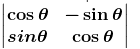⇒ |A| = cos θ × cos θ – (–sin θ) x sin θ

|A| = cos2θ + sin2θ

We know that cos2θ + sin2θ = 1

|A| = 1

(iii) Given⇒ |A| = cos15° × cos75° + sin15° x sin75°

We know that cos (A – B) = cos A cos B + Sin A sin B

By substituting this we get, |A| = cos (75 – 15)°

|A| = cos60°

|A| = 0.5

(iv) Given⇒ |A| = (a + ib) (a – ib) – (c + id) (–c + id)

= (a + ib) (a – ib) + (c + id) (c – id)

= a2 – i2 b2 + c2 – i2 d2

We know that i2 = -1

= a2 – (–1) b2 + c2 – (–1) d2

= a2 + b2 + c2 + d2

3. Evaluate: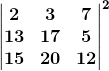Solution:

Since |AB|= |A||B|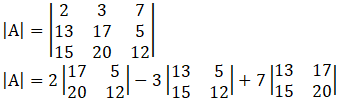= 2(17 × 12 – 5 × 20) – 3(13 × 12 – 5 × 15) + 7(13 × 20 – 15 × 17)

= 2 (204 – 100) – 3 (156 – 75) + 7 (260 – 255)

= 2×104 – 3×81 + 7×5

= 208 – 243 +35

= 0

Now |A|2 = |A|×|A|

|A|2= 0

4. Show that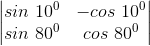Solution:

GivenLet the given determinant as A

Using sin (A+B) = sin A × cos B + cos A × sin B

⇒ |A| = sin 10° × cos 80° + cos 10° x sin 80°

|A| = sin (10 + 80)°

|A| = sin90°

|A| = 1

Hence Proved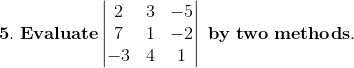Solution:

Given,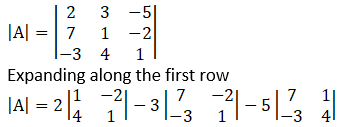= 2(1 × 1 – 4 × (–2)) – 3(7 × 1 – (–2) × (–3)) – 5(7 × 4 – 1 × (–3))

= 2(1 + 8) – 3(7 – 6) – 5(28 + 3)

= 2 × 9 – 3 × 1 – 5 × 31

= 18 – 3 – 155

= –140

Now by expanding along the second column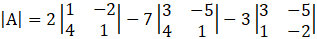= 2(1 × 1 – 4 × (–2)) – 7(3 × 1 – 4 × (–5)) – 3(3 × (–2) – 1 × (–5))

= 2 (1 + 8) – 7 (3 + 20) – 3 (–6 + 5)

= 2 × 9 – 7 × 23 – 3 × (–1)

= 18 – 161 +3

= –140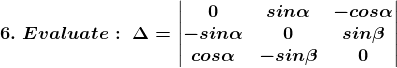Solution:

Given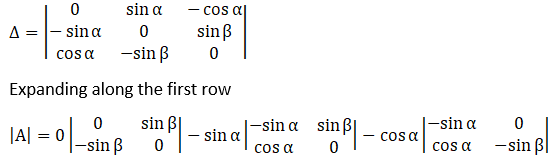⇒ |A| = 0 (0 – sinβ (–sinβ)) –sinα (–sinα × 0 – sinβ cosα) – cosα ((–sinα) (–sinβ) – 0 × cosα)

|A| = 0 + sinα sinβ cosα – cosα sinα sinβ

|A| = 0